#### Select Case Statement in Excel Vba

The Select Case statement is a decision-making statement in Excel VBA that allows you to execute different actions based on the value of an expression. It is a more flexible and readable alternative to the If statement.

In this tutorial, you will learn how to use the Select Case statement in Excel VBA. You will also learn about the advantages and disadvantages of using the Select Case statement.

## Select Case Formula

The syntax of the Select Case statement is as follows:

```Select Case
Case
Do something
Case
Do something
Case
Do something
Case Else
Do something else
End Select
```

The expression is the value that you want to test. The value1, value2, etc. are the possible values of the expression. The statements are the actions that you want to execute for each value.

The Case Else statement is optional. It is used to execute a set of statements if none of the other cases are true.

## The code

Click on the Developer tab and select “View Code”.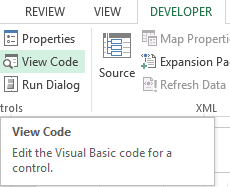A new window (Visual Basic Editor) will open which will have a dialog box in the center.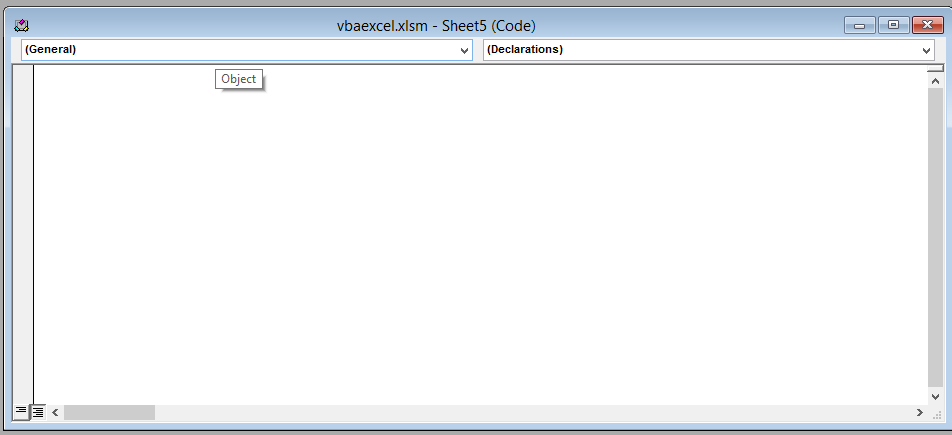Write the following line of code in the dialog box.

```Sub selectcase ()
Select Case Range ("A1").Value
Case 100
Range ("B1").Value = 50
Case 150
Range ("B1").Value = 40
Case Else
Range ("B1").Value = 0
End Select
End Sub```

In this code we are simply changing the value in cell B if there is a certain value in cell A. If the user entered 100 in cell A1 than the value in cell B1 will be 50. If the user changes the value in cell A1 to 150, then the value in cell B1 would be 40.

“Case Else” means that if none of the above conditions are true (meaning if the values in cell A1 aren’t 100, 150) then the value in cell B1 would be 0. End Select is used to end the SELECT CASE statement.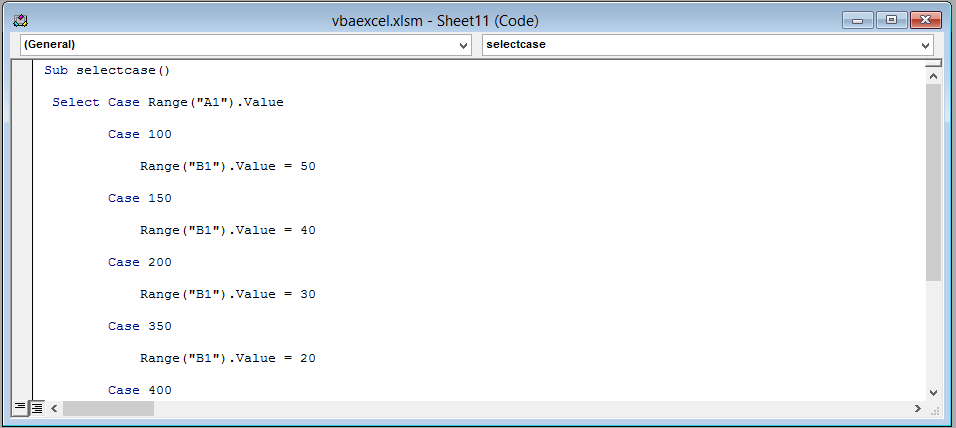## The result

This is the result if the user has entered 100 in cell A1.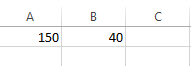This is the result if the user has entered 150 in cell A1.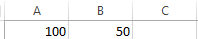This is the result if the user has entered any value in cell A1 except 100 or 150.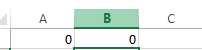## Advantages of using the Select Case statement:

The Select Case statement is more flexible than the If statement. You can test for multiple values with the Select Case statement, while the If statement can only test for one value.

The Select Case statement is easier to read and understand than the If statement.

## Disadvantages of using the Select Case statement:

The Select Case statement can be slower than the If statement if there are many cases.

The Select Case statement can be more difficult to debug than the If statement.

If you are looking for a way to execute different actions based on the value of an expression, then the Select Case statement is a good option.